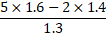# Quiz Discussion

$$\frac{{{{\left( {0.013} \right)}^3} + 0.000000343}}{{{{\left( {0.013} \right)}^2} - 0.000091 + 0.000049}} = \,?$$ = ?

Course Name: Quantitative Aptitude

• 1] 0.002
• 2] 0.020
• 3] 0.021
• 4] 0.023
##### Solution
No Solution Present Yet

#### Top 5 Similar Quiz - Based On AI&ML

Quiz Recommendation System API Link - https://fresherbell-quiz-api.herokuapp.com/fresherbell_quiz_api

# Quiz
1
Discuss

Which of the following is equal to 3.14 x 106 ?

• 1] 314
• 2] 3140
• 3] 3140000
• 4] None of these
##### Solution
2
Discuss

[(?)2 + (18)2] ÷ 125 = 3.56

• 1] 11
• 2] 12
• 3] 14
• 4] 15
• 5] None of these
##### Solution
3
Discuss

Which of the following has fractions in ascending order ?

• 1]

$$\frac{2}{3},\frac{3}{5},\frac{7}{9},\frac{9}{{11}},\frac{8}{9}$$

• 2]

$$\frac{3}{5},\frac{2}{3},\frac{9}{11},\frac{7}{{9}},\frac{8}{9}$$

• 3]

$$\frac{3}{5},\frac{2}{3},\frac{7}{9},\frac{9}{{11}},\frac{8}{9}$$

• 4]

$$\frac{8}{9},\frac{9}{11},\frac{7}{9},\frac{2}{{3}},\frac{3}{5}$$

• 5]

$$\frac{8}{9},\frac{9}{11},\frac{7}{9},\frac{3}{{5}},\frac{2}{3}$$

##### Solution
4
Discuss

The number 0.121212 ... in the form p/q is equal to

• 1]

4/11

• 2]

2/11

• 3]

4/33

• 4]

2/33

##### Solution
5
Discuss

The number 0.121212 ..... in the form p/q is equal to :

• 1]

2/11

• 2]

4/11

• 3]

2/33

• 4]

4/33

##### Solution
6
Discuss

Solve 21.5/5 + 21/6 - 13.5/15 = {(?)1/3 /4} +17/30

• 1] 2
• 2] 8
• 3] 512
• 4] 324
• 5] None of these
##### Solution
7
Discuss

0.04 x 0.0162 is equal to:

• 1] 6.48 x 10-3
• 2] 6.48 x 10-4
• 3] 6.48 x 10-5
• 4] 6.48 x 10-6
##### Solution
8
Discuss

The numerator of a fraction is decreased by 25% and the denominator is increased by 250%. If the resultant fraction is 6/5, what is the original fraction?

• 1]

22/5

• 2]

24/5

• 3]

27/6

• 4]

28/5

• 5]

30/11

##### Solution
9
Discuss

The least among the following is:

• 1]

0.2

• 2]

1 ÷ 0.2

• 3]

$$0.\overline 2$$

• 4]

(0.2)2

##### Solution
10
Discuss= ?

• 1] 0.4
• 2] 1.2
• 3] 1.4
• 4] 4
# Quiz